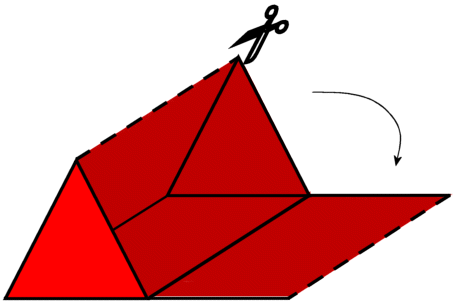Subject: surface area of a triangular prism Name: Amanda Who are you: Student What is the formula for the surface area of a triangular prism? Hi Amanda, The surface area of the prism is the sum of the areas of the faces of the prism. Think about cutting along the edges of your prism, so that you can unfold it into a set of triangles and rectangles or parallelograms. If your triangular prism is a right triangular prism, then the surface is composed of triangles and rectangles. If it is an oblique triangular prism, then the surface is composed of triangles and parallelograms.Do you know the formulae for the area of a triangle and a parallelogram? Haley